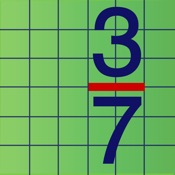# 3000+ Fractions - Lumos Educational App Store1
Price -\$1.28
\$1.28

#### DESCRIPTION:

3000+ Fractions consists of over 3000 fraction questions. Reviewed and highly recommended by educationalappstore.com. The questions progress in difficulty and are suitable for learners in the age range 10 to 16+. Please be aware that this App is for the later stages of the primary and secondary education mathematics curriculum. It is NOT aimed at pre-school and elementary levels. " 3000+ Fractions is a great math app to help and support students aged 10 to 16+ to master all aspects of fractions. " - funeducationalapps.com The common core elements covered are as follows: -

#### OVERVIEW:

3000+ Fractions is a free educational mobile app By Lucyalex Limited.It helps students in grades 3,4,5 practice the following standards 3.NF.3,4.NF.C.5,5.NF.B.3.

This page not only allows students and teachers download 3000+ Fractions but also find engaging Sample Questions, Videos, Pins, Worksheets, Books related to the following topics.

1. 3.NF.3 : Explain equivalence of fractions in special cases, and compare fractions by reasoning about their size. (Grade 3 expectations in this domain are limited to fractions with denominators 2, 3, 4, 6, and 8.).

2. 4.NF.C.5 : Express a fraction with denominator 10 as an equivalent fraction with denominator 100, and use this technique to add two fractions with respective denominators 10 and 100. For example, express 3/10 as 30/100 and add 3/10 + 4/100 = 34/100. (Students who can generate equivalent fractions can develop strategies for adding fractions with unlike denominators in general. But addition and subtraction with unlike denominators in general is not a requirement at this grade.) (Grade 4 expectations in this domain are limited to fractions with denominators 2, 3, 4, 5, 6, 8, 10, 12, and 100.).

3. 5.NF.B.3 : Interpret a fraction as division of the numerator by the denominator (a/b = a ÷ b). Solve word problems involving division of whole numbers leading to answers in the form of fractions or mixed numbers, e.g., by using visual fraction models or equations to represent the problem. For example, interpret 3/4 as the result of dividing 3 by 4, noting that 3/4 multiplied by 4 equals 3 and that when 3 wholes are shared equally among 4 people each person has a share of size 3/4. If 9 people want to share a 50-pound sack of rice equally by weight, how many pounds of rice should each person get? Between what two whole numbers does your answer lie?.

3
4
5

3.NF.3
4.NF.C.5
5.NF.B.3

#### ADDITIONAL INFORMATION:

Developer: Lucyalex Limited

Software Version: 1.0.3

Category: Education

### RELATED APPSEdSearch WebSearch